SPSS TUTORIALS BASICS ANOVA REGRESSION FACTOR CORRELATION

# SPSS Variable Types and Formats

Understanding SPSS variable types and formats allows you to get things done fast and reliably. Getting a grip on types and formats is not hard if you ignore the very confusing information under variable view. This tutorial will put you on the right track.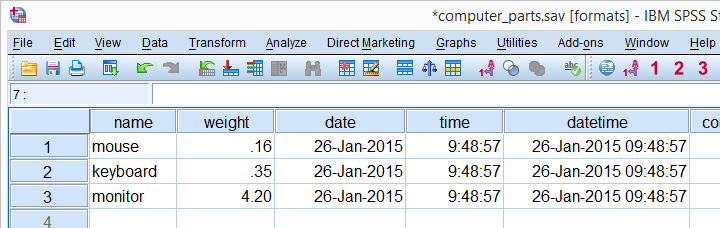## SPSS Variable Types

SPSS has 2 variable types:

• Numeric variables contain only numbers and are suitable for numeric calculations such as addition and multiplication.
• String variables may contain letters, numbers and other characters. You can't do calculations on string variables -even if they contain only numbers.

There are no other variable types in SPSS than string and numeric. However, numeric variables have several different formats that are often confused with variable types. We'll see in a minute how variable view puts users on the wrong track here.
The only way to change a string variable to numeric or reversely is ALTER TYPE. However, there's several ways to make a numeric copy of a string variable or reversely. We'll get to those in a minute.

## So What's Better: String or Numeric?

The simplest rule of thumb is that only nominal variables with many categories
should be string variables in SPSS.
Examples are names of people, email addresses, passport numbers and so on. Although such variables can be useful, we don't usually analyze them.
We do sometimes analyze nominal variables with few categories -such as nationality, blood group or profession. If these are string variables, they may or may not cause trouble. For example, the independent variable for ANOVA may or may not be a string variable depending on the exact command you use for it.Precisely, UNIANOVA does and ONEWAY does not accept string variables as factors.
You may get away by leaving such variables as strings. However, copying them into numeric variables makes sure you'll avoid all trouble. A decent way to do so is AUTORECODE. For converting metric string variables -holding just numbers- into numeric variables, see SPSS Convert String to Numeric Variable.

## Determining SPSS Variable Types

So how do we know if a variable is string or numeric? In SPSS versions 24 and higher, tiny icons in front of variable names tell us the variable type, format and even measurement level. The icon for “nominal” may contain a tiny “a” which indicates it's a string variable.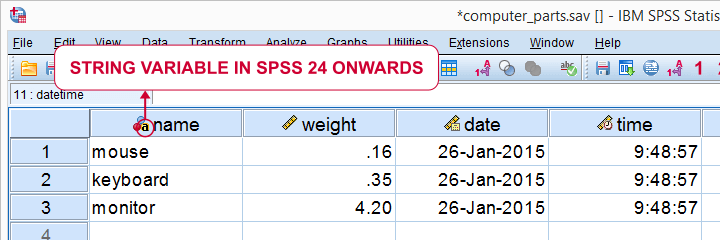For SPSS versions 23 and earlier, we'll inspect our variable view and use the following rule:

• if Type says “String”, you're dealing with a string variable;
• if Type does not say “String”, you're dealing with a numeric variable.SPSS suggests that “Date” and “Dollar” are variable types as well. However, these are formats, not types. The way they are shown here among the actual variable types (string and numeric) is one of SPSS’ most confusing features.

## SPSS Variable Formats - Introduction

Let's now have a look at the data in data view as shown the screenshot below. We'll briefly describe the kinds of variables we see.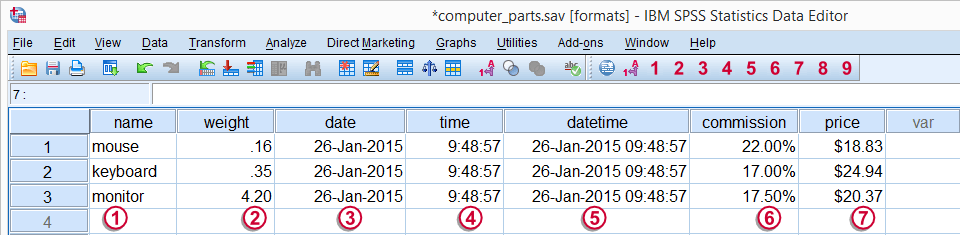Regarding these data, we stated earlier thatis a string variable andthrough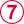are numeric variables and contain only numbers.

However, values such as “26-jan-2015” sure don't look like numbers, do they? This is because SPSS can display numbers in very different ways. These ways of displaying data values are referred to as variable formats.

## Determining SPSS Variable Formats

As we saw earlier, “Type” under variable view shows a confusing mixture of variable types and formats. We'll see the actual formats by running display dictionary. Part of the result is shown by the screenshot below.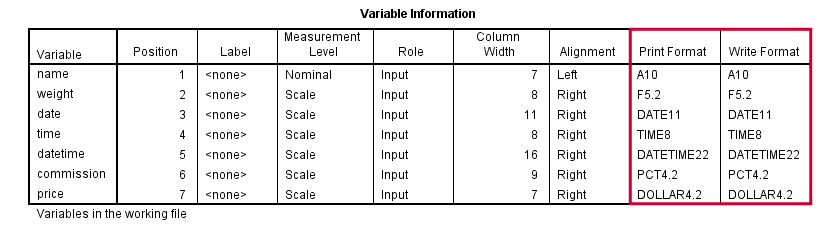SPSS distinguishes print and write formats but we don't bother about this distinction. SPSS variable formats consist of two parts. One or more letters indicate the format family. Most of them speak to themselves, except for the first two variables:

• A (“Alphanumeric”) is the usual format for string variables;
• F, (“Fortran”) indicates a standard numeric variable.

Formats end with numbers, indicating the number of characters to be shown. If a period is present, the number after the period indicates the number of decimal places to be displayed. The figure below illustrates these points.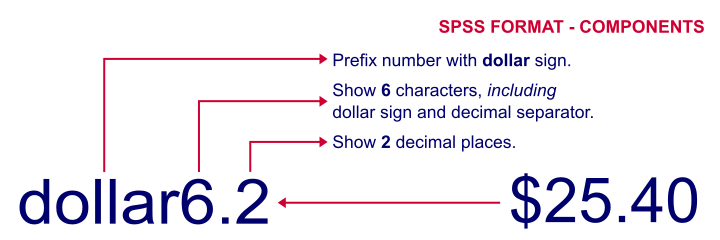## SPSS Common Variable Formats

The figure below now summarizes some common variable types and formats we'll encounter in SPSS.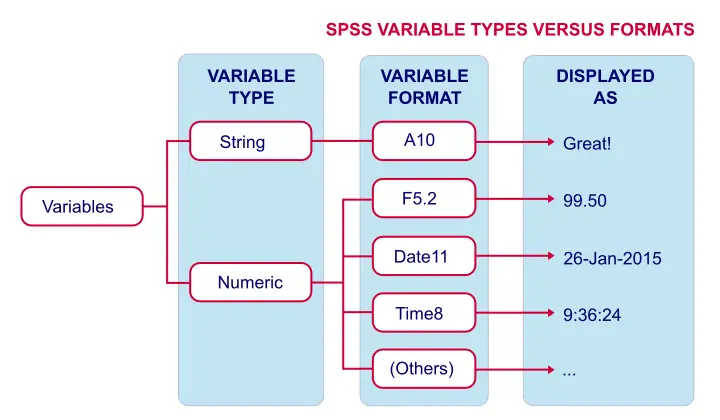## Setting Variable Formats in SPSS

You can set variable formats for numeric variables with the FORMATS command. For example, formats weight (f4.3). shows weight with 3 decimal places. Doing so affects the output you create: most tables will add an extra decimal place for weight as well. If you'd like to see this for yourself, run the syntax below and compare the 2 resulting tables.

*Show 2 decimal places for weight and run descriptives.

formats weight(f3.2).

descriptives weight.

*Show 3 decimal places for weight and run descriptives.

formats weight(f4.3).

descriptives weight.

*Note that second output table shows more decimal places.

Keep in mind that changing variable formats does not change your data in any way. The actual values are still the exact same numbers. They are merely displayed differently.

## Variable Types and Formats - Why Bother?

Basically, “what you see is not what you get” in data view. For example, we see \$20.37 but the actual value is just 20.37. So we can identify products costing \$20,- or more by running the syntax below: compute expensive = (price >= 20). We don't include the dollar sign in our syntax. Although SPSS shows a dollar sign in data view, the actual values are just numbers and these are what the syntax acts upon.

Or let's say we'd like to add 30 days to our date variable. We could do so by running compute newdate = datesum(date,30,'days'). The resulting values are 13644236937.72. These are the correct numbers but they'll display as readable dates only after running something like formats newdate (date11). Another reason for bothering about variable formats is setting decimals places for output tables. For SPSS version 22 onwards, OUTPUT MODIFY does the trick as shown below.

*Create basic descriptives table.

descriptives weight.

*Set 2 decimal places (format = f3.2) for mean and SD (columns 4 and 5).

output modify
/select tables
/tablecells select = [position(4) position(5)] selectdimension = columns format = 'f3.2'.

In a similar vein, CTABLES allows choosing different formats for different statistics in your output.

*Create descriptives table with different decimal places for different statistics.

ctables
/table commission [count 'N' f3 Minimum pct3 Maximum pct3 mean 'Mean' pct4.1 stddev 'SD' pct4.1].

## Final Notes

This tutorial was somewhat theoretical but it has a lot of practical consequences. I hope you found it helpful.

•